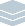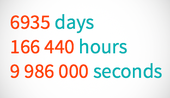Wolfram Archive
Wolfram Programming Lab is a legacy product.
All the same functionality and features, including access to Programming Lab Explorations, are available with Wolfram|One.
Start programming now. »
Try it now »
(no sign-in required)# Age in Days, Hours, etc.

Enter a date:

DateObject gives you an exact date that you can do computations with:

DateObject["November 16, 1998"]

It understands most common date formats:

DateObject["11/16/98"]
DateObject["16.11.98"]
DateObject["1998 Nov 16"]

DateObject["November 16, 1998"]

Run the code to compute the number of days from now back to a date. Try other dates:

Now gives you the date and time at the instant you run it:

Now

Subtract a date from Now to find the number of days back to the date:

Now -DateObject["November 16, 1998"]

Now - DateObject["November 16, 1998"]

Round to whole days. Try other dates:

Round rounds decimal numbers to integers:

Round[4.56]

Round a date computation to get an answer in whole days:

Round[Now - DateObject["November 16, 1998"]]

Round[Now - DateObject["November 16, 1998"]]

Convert to minutes. Try other dates, like your birthday (to find out how many minutes old you are):

UnitConvert converts between different units, like grams and pounds, or days and minutes.

This gives an answer in days:

Round[Now - DateObject["November 16, 1998"]]

Convert the result to minutes:

UnitConvert[Round[Now - DateObject["November 16, 1998"]], "Minutes"]

Round[UnitConvert[Now - DateObject["November 16, 1998"], "Minutes"]]

Spell out the result. Try other dates:

The result of a date calculation has both a value (the number) and a unit (min):

Round[UnitConvert[Now - DateObject["November 16, 1998"], "Minutes"]]

Use QuantityMagnitude to get just the value:

QuantityMagnitude[ Round[UnitConvert[Now - DateObject["November 16, 1998"], "Minutes"]]]

IntegerName gives the spelled-out names of numbers:

IntegerName

Spell out the result of a date calculation:

IntegerName[ QuantityMagnitude[ Round[UnitConvert[Now - DateObject["November 16, 1998"], "Minutes"]]]]

IntegerName[ QuantityMagnitude[ Round[UnitConvert[Now - DateObject["November 16, 1998"], "Minutes"]]]]

Get the result in seconds. Try other units, like "Hours", "Weeks" or "Months":

IntegerName[ QuantityMagnitude[ Round[UnitConvert[Now - DateObject["November 16, 1998"], "Seconds"]]]]

Share ItMake a website that tells you how many days old you are. Try making a site that tells you how many seconds old you are:

Deploy the days old calculation to make a website where you can enter a birthdate and find out how many days old you are:

CloudDeploy[FormFunction[{"birthdate" -> "Date"}, Round[Now - DateObject[#birthdate]] &, "PNG" ], Permissions -> "Public"]

Click the link in the output to visit the site.

Format the output more nicely using Row:

CloudDeploy[FormFunction[{"birthdate" -> "Date"}, Row[{"You are ", QuantityMagnitude[Round[Now - DateObject[#birthdate]]], " days old."}] &, "PNG" ], Permissions -> "Public"]

Make the text larger:

CloudDeploy[FormFunction[{"birthdate" -> "Date"}, Style[Row[{"You are ", QuantityMagnitude[Round[Now - DateObject[#birthdate]]], " days old."}], 48] &, "PNG" ], Permissions -> "Public"]

Click the link in the output to visit the site.